# Problem of the Month (August 2002)

The Partridge Problem, originated by Bob Wainwright, is now well known in packing circles. Since 13 + 23 + . . . + n3 = [ n(n+1)/2 ]2, the area of 1 square of side 1, 2 squares of side 2, . . . , and n squares of side n fit exactly inside a square of side n(n+1)/2. It turns out the smallest value of n for which this packing exists is n=8. (This was originally found by Bill Cutler, William Marshall, Michael Reid, and Nob Yoshigahara.) We call this the partridge number of the square. The packing is shown below: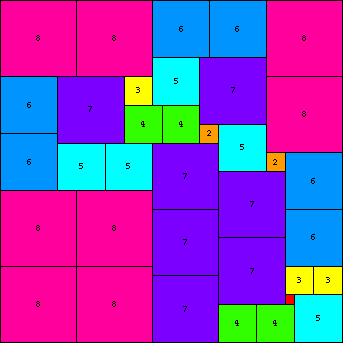What are the partridge numbers of other polyominoes, or other shapes?

Bill Cutler found the partridge number of a 2×1 rectangle is 7: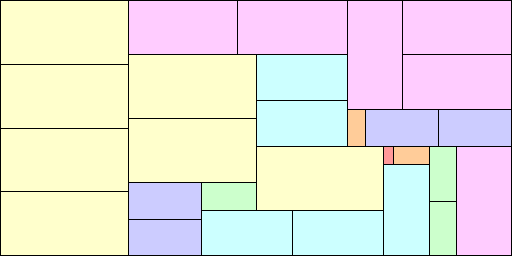He also found the partridge number of a 3×1 rectangle is 6. Ed Pegg reminded me that Andrew Clarke and Joseph DeVincentis found that this is easily hand-solved if one takes into parity issues: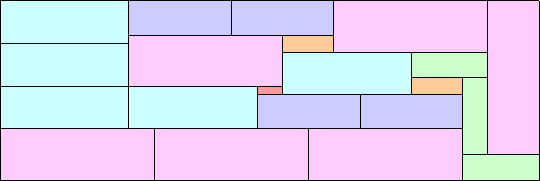Patrick Hamlyn and his marvelous computer packing program found some amazing packings. He and Bill Cutler counted the number of partridge packings of squares 1-8 in a 36×36 square and came up with 2332. This implies that the partridge number of all parallelograms is no more than 8.

He found that the partridge number of the 4×1 rectangle is 7: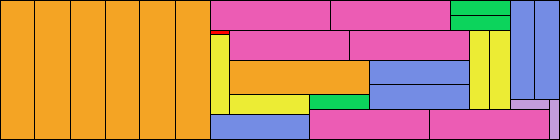He found the partridge number of the 5×1 rectangle is 8. We can get such packings by stretching partridge packings of squares, but here's one that involves some vertical rectangles as well: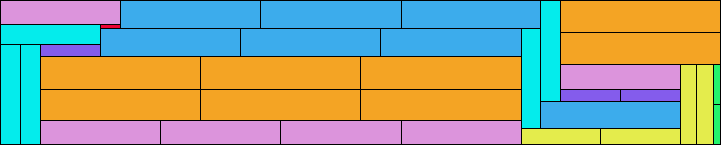He also found the partridge numbers of the 3×2 rectangle, 4×3 rectangle, and 8×3 rectangle are 7: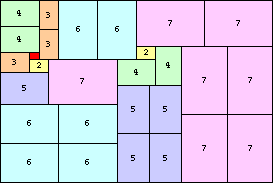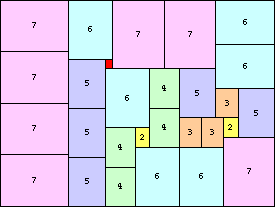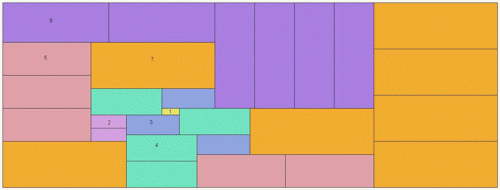Here is a shape with partridge number 4 by Mike Reid. This can be stretched along either diagonal axis to give other shapes with partridge number 4. Another description of these trapezoids is that they have bases of lengths 3 and 6, and at least one side of length 8.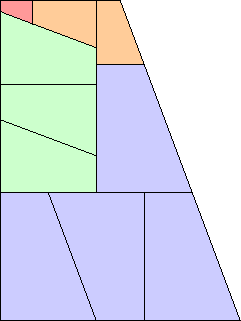William Marshall found that a packing exists for n=9 for the equilateral triangle, and Patrick Hamlyn confirmed that this was the smallest possible. This implies that the partridge number of all triangles is no more than 9.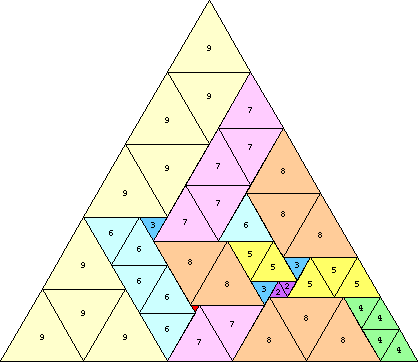And Patrick Hamlyn found that the partridge number of the triamond is 5: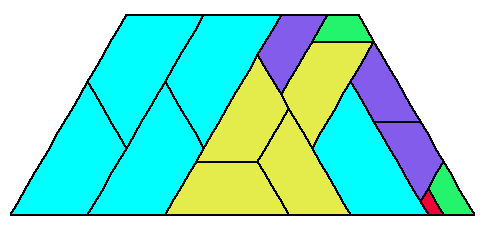He found that the partridge number of the 30-60-90 triangle is 4: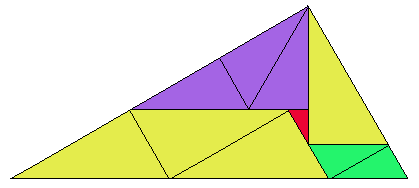He found that the partridge number of the 45-45-90 triangle is 8. This implies that the partridge number of all right triangles is no more than 8.He also found that the partridge number of this trapezoid which is the union of 3 30-60-90 triangles is 6: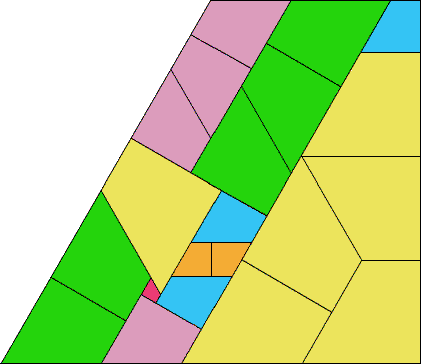I found another infinite family of trapezoids (bases 1 and 2 and another side of length 2) with partridge number 6: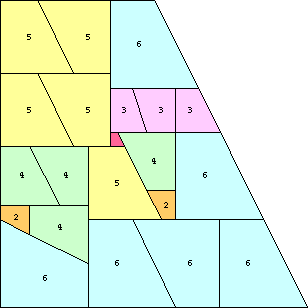In 2020, George Sicherman sent me this packing of half a domino with partridge number 6: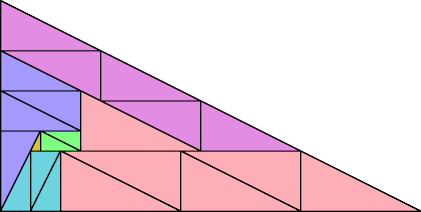Philippe Fondanaiche generalized and found tilings of a square by 1 through n squares of size 1 through n, but not necessarily in that order. He did this for n=7 and n=8 . He conjectures that the size of the smallest square that can be packed with n different sized squares is 1 +(n+1)√(n(n+2)/12). He also notes that if there is a partridge packing of 2n different squares, there is also a partridge packing of 2n+1 different squares. Mike Reid points out the same is true of parallelograms and triangles.

Here is a catalog of the the small squares which can be packed with 1 through n squares of size 1 through n.

## Distinct Square Packings

n Sizes of Square
11
511, 12, 13
614, 16, 18
719, 20, 21, 22, 23, 24, 25, 26
824, 25, 26, 27, 28, 29, 30, 31, 32, 36

Here are some small triangles which can be packed with 1-n triangles of size 1-n.

## Distinct Triangle Packings

n Sizes of Square
11
512, 13
614, 16

We can try to pack the partridge squares into rectangles as well. William Marshall found the following packings, which Patrick Hamlyn showed to be the smallest possible.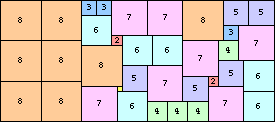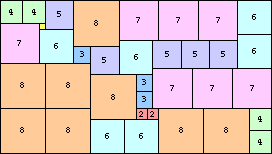You can order some of these partridge tilings in beautiful acrylic from Kadon. They make excellent puzzles.

If you can extend any of these results, please e-mail me. Click here to go back to Math Magic. Last updated 9/12/02.